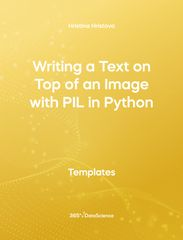# Resources

Save hours of browsing through the Internet and accelerate your workflow with code-ready templates for your programming projects. Personally crafted by 365 Data Science instructors, these templates will bring a surprising level of efficiency to your work process.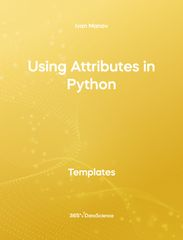Templates python
###### Using Attributes in Python

This template shows  how to use attributes for gathering information about different Objects - in particular - pandas Series. Some other related topics you might be interested in are Using Pandas Methods for Working with Series Objects in Python, Creating DataFrames in Python, Delivering an Array with the Unique Values from a Dataset in Python, Converting Series into Arrays in Python, and Ordering the Rows from a Data Table According to the Values in a Column in Python. The Using Attributes in Python template is among the topics covered in detail in the 365 Program.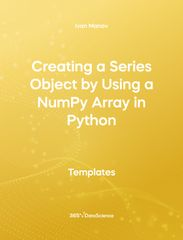Templates python
###### Creating a Series Object by Using a NumPy Array in Python

This template shows  how to convert a NumPy array into a Series. First, you import the Pandas and NumPy libraries, after which you create an array containing four integer values. Then you turn the array into a series and finally you check the type of the object. Some other related topics you  might be interested in are  Using Attributes in Python, Indexing in Pandas Python, Position-Based and Label-Based Indexing in Python, and Dealing with Indexing in Python. The creating a Series Object by Using a NumPy Array in Python template is among the topics covered in detail in the 365 Program.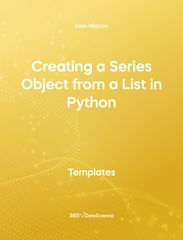Templates python
###### Creating a Series Object from a List in Python

This template shows how to convert a list object into a Series in the popular Pandas library. Some other related topics you might be interested in are Creating a Series Object by Using a NumPy Array in Python, Using Pandas Methods for Working with Series Objects in Python, Obtaining Descriptive Statistics about the Data in Python, Delivering an Array with the Unique Values from a Dataset in Python, and Ordering the Rows from a Data Table According to the Values in a Column in Python. The Creating a Series Object from a list in Python template is among the topics covered in detail in the 365 Program.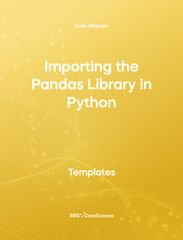Templates python
###### Importing the Pandas Library in Python

This template demonstrates how to import the pandas library in Python- a popular open-source library that provides high-performance structures and data analysis tools. Some other related topics you might be interested in are Indexing in Pandas Python, Using Attributes in Python, Using Pandas Methods for Working with Series Objects in Python, and Creating DataFrames in Python. The Importing the Pandas Library in Python is among the topics covered in detail in the 365 Program.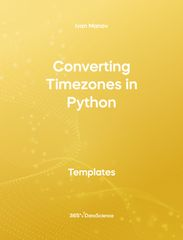Templates python
###### Converting Timezones in Python

This free open-access template shows how to convert date and time values between the different time zones in Python. Some other related topics you might be interested in are Local Time and Universal Time in Python, Importing the Pandas Library in Python, Creating a Series Object by Using a NumPy Array in Python, and Using Attributes in Python. The Converting Timezones in Python template is among the topics covered in detail in the 365 Program.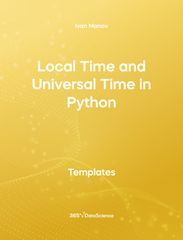Templates python
###### Local Time and Universal Time in Python

This is an open-access template demonstrating how to estimate the local time and compare it to universal time in Python. Some other related topics you might be interested in are Converting Timezones in Python, Converting between Timezones in DataFrames, Importing the Pandas Library in Python, and Creating a Series Object from a List in Python. The Local Time and Universal Time in Python template is among the topics covered in detail in the 365 Program.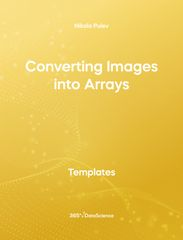Templates python
###### Converting Images into Arrays

The following template teaches you one way to convert an image file to an array (tensor). This is extremely useful in ML and Computer Vision, as these fields require images as data, however, the algorithms can only work with numbers and arrays. Some other related topics you might be interested in are Tensorboard - Tracking Metrics in Python, Tensorboard - Confusion Matrix in Python, Tensorboard - Tuning Hyperparameters in Python, and A Simple CNN Network - Convolutional Layer in Python. The Converting Images into Arrays in Python is among the topics covered in great detail in the 365 Data Science Program.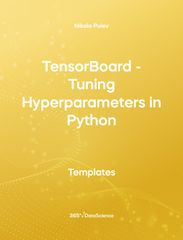Templates python
###### TensorBoard - Tuning Hyperparameters in Python

This template demonstrates how one can tune the hyperparameters of their network model using TensorBoard. Hyperparameter tuning is important aspect of Machine Learning and being able to do it automatically can be a time saver. TensorBoard provides other visualization options, as well. Some other related topics you might be interested in are Dropout in Python, L2 Regularization and Weight Decay in Python, Converting Images into Arrays, and A Common CNN Architecture in Python. The TensorBoard – tuning Hyperparameters in Python.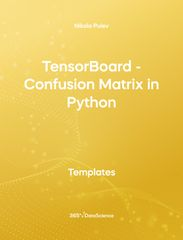Templates python
###### TensorBoard – Confusion Matrix in Python

The confusion matrix is an essential tool when trying to solve classification problems. There are many different ways to construct such a matrix. In the following template we show you how one can create and visualize the confusion matrix with the help of TensorBoard and sklearn. Some other related topics you might be interested in are TensorBoard - Tuning Hyperparameters in Python, Converting Images into Arrays, L2 Regularization and Weight Decay in Python, and Dropout in Python. The TensorBoard template is among the topics covered in detail in the 365 Program.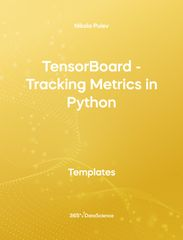Templates python
###### TensorBoard - Tracking Metrics in Python

The following is a program used to demonstrate how to log different metrics in Tensorboard for visualization later. An example CNN network is used. The TensorBoard callback is defined to log the loss function and accuracy during training. Then, the extension is loaded in order to visualize these metrics. Some other related topics you might be interested in are TensorBoard - Confusion Metrics in Python, TensorBoard - Tuning Hyperparameters in Python, Converting Images into Arrays. The TensorBoard - Tracking Metrics in Python template is among the topics covered in the 365 Data Science Program.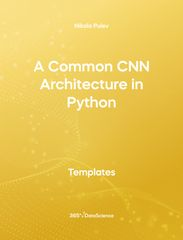Templates python
###### A Common CNN Architecture in Python

Convolutional Neural Networks are a powerful choice for problems and datsets involving images. However, they can grow to become so big, that training it on a normal system takes too long. So, the following template shows a particular network architecture that can be very effective for most problems, but is also small enough to be trained quickly. Some other related topics you might be interested in are Pooling Layers in Python, Tensorboard - Tracking Metrics in Python, Tensorboard - Confusion Metrics in Python, and Tensorboard - Tuning Hyperparameters in Python. The Common CNN Architecture in Python template is among the topics covered in detail in the 365 Program.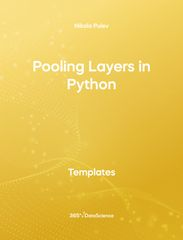Templates python
###### Pooling Layers In Python

Pooling Layers are an important part of a Convolutional Neural Network (CNN). That's why, the following template demonstrates how one can add a MaxPooling layer to the network architecture, as well as discuss the important parameters that need to be considered and included. Some other related topics you might be interested in are Simple CNN Network - Convolutional Layer in Python, A Common CNN Architecture in Python, TensorBoard - Tracking Metrics in Python, and Tensorboard - Tuning Hyperparameters in Python. The Pooling Layers in Python template is among the topics covered in detail in the 365 Program.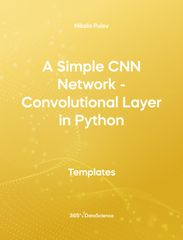Templates python
###### A Simple CNN Network - Convolutional Layer in Python

This template demonstrates how one can add convolutional layers to our network in order to create a Convolutional Neural Network (CNN)..  Some other related topics you might be interested in are Pooling Layers in Python, A Common CNN Architecture in Python, Tensorboard - Tracking Metrics in Python, and Tensorboard - Tuning Hyperparameters in Python. The template is among the topics covered in detail in the 365 Program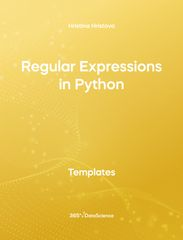Templates python
###### Regular Expressions in Python

In this template you will find a list of the most commonly used regular expressions as well as a link to the Python documentation website, where the full list is stored. We will start with a simple example demonstrating the function of the compile(), match(), and search() methods. After that, more complicated regular expressions are constructed. Some other related topics you might be interested in are String formatting in Python, Bubble Sort in Python, Linear search in Python, Binary Search in Python. The Regular Expressions in Python template is among the topics covered in detail in the 365 Data Science Program.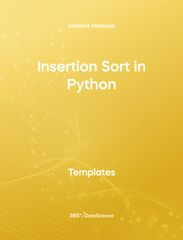Templates python
###### Insertion Sort in Python

The following template demonstrates how to implement an insertion sort function in Python. Some other related topics you might be interested in are Bubble sort in Python, Linear search in Python, Binary search in Python, and Sets and operations with sets in Python. The Insertion Sort in Python is among the topics covered in detail in the 365 Data Science Program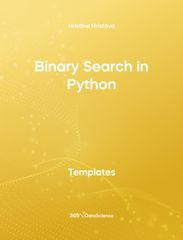Templates python
###### Binary Search in Python

The following notebook demonstrates how to implement a binary search function(also knows as half interval) in Python. Some other related topics you might be interested in are Bubble sort in Python, Linear search in Python, and Sets and operations with sets in Python. The Binary Search in Python is among the topics covered in detail in the 365 Data Science Program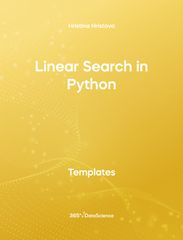Templates python
###### Linear Search in Python

The following Jtemplate demonstrates how to implement a linear search function in Python. Some other related topic you might be interested in Bubble sort in Python, Insertion sort in Python, Binary search in Python, Sets and operations with sets in Python are Bubble Sort in Python, Insertion Sort in Python, Binary Search in Python, Sets and Operations with Sets in Python. The Linear Search in Python template is among the topics covered in detail in the 365 Data Science Program.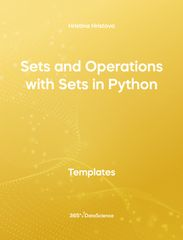Templates python
###### Sets and Operations with Sets in Python

The following template demonstrates the difference between lists and sets in Python, and includes examples. Some other related topics you might be interested in are Linear search in Python, Binary search in Python, Insertion sort in Python, Bubble sort in Python. The Set and Operations with Sets in Python Template is among the topics covered in detail in the 365 Data Science Program.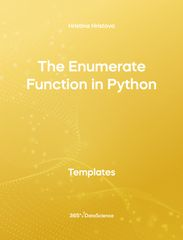Templates python
###### The Enumerate Function in Python

The following notebook demonstrates how to iterate through a list more effectively using the enumerate() built-in function in Python. Some other related topics you might be interested in are The zip function in Python, Defining functions in Python - the Fibonacci sequence,  Recursion in Python - the Fibonacci sequence and Defining Classes in Python. The Enumerate Function in Python template is among the topics covered in detail in the 365 Data Science Program.